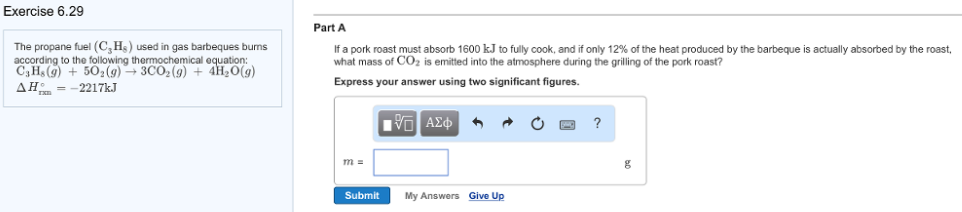# Problem: The propane fuel (C3H8) used in gas barbeques buns according to the following thermochemical equation: C3H8 (g) + 5O2 (g) → 3CO2 (g) + 4H2O (g)    ΔH°rxn = -2217kJ If a pork roast must absorb 1600 kJ to fully cook, and if only 12% of the heat produced by the barbeque is actually absorbed by the roast, what mass of CO2 is emitted into the atmosphere during the grilling of the pork roast? Express your answer using two significant figures.

###### FREE Expert Solution
96% (373 ratings)###### Problem Details

The propane fuel (C3H8) used in gas barbeques buns according to the following thermochemical equation:

C3H(g) + 5O2 (g) → 3CO2 (g) + 4H2O (g)    ΔH°rxn = -2217kJ

If a pork roast must absorb 1600 kJ to fully cook, and if only 12% of the heat produced by the barbeque is actually absorbed by the roast, what mass of CO2 is emitted into the atmosphere during the grilling of the pork roast? Express your answer using two significant figures.